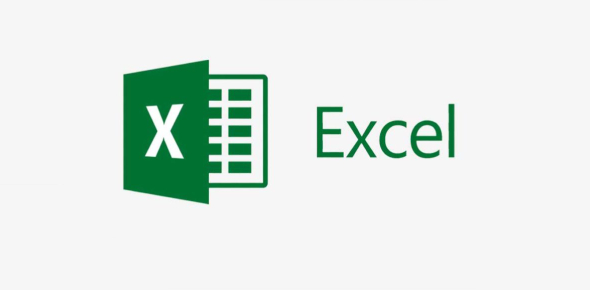# MS Excel Basic Knowledge! Ultimate Trivia Quiz

10 Questions | Total Attempts: 1171Settings.

• 1.
The intersection of a row and a column is called _________.
• 2.
The cell labeled D8 refers to
• A.

Row D, column 8

• B.

Column D, row 8

• C.

Function key 8

• 3.
What do these buttons do?
• A.

Fill text with the fill handles

• B.

Sort data

• C.

Create a chart

• 4.
Which formula is correct for computing the average?
• A.

Average(A1..A4)

• B.

=avg(A1:B5)

• C.

=average(B1:B4)

• 5.
What does the button below do?
• A.

Decrease decimal

• B.

• C.

Increase decimal

• 6.
What is wrong with the cell to the right of Dogs?
• A.

The formula has an error

• B.

The row is too short to show the number

• C.

The column is too narrow to show all the digits

• 7.
When you are typing a formula into a cell, what is the first thing you must enter?
• 8.
If you press the return key, the cell accepts the data and moves to the next _______.
• 9.
If you press the tab key, the cell accepts the data and moves to the next ________.
• 10.
Jack wants to select the data in the A column, and the C column. What key should he hold down to select cells that are not right next to each other?
• A.

Command

• B.

Control

• C.

Shift

Related TopicsBack to top